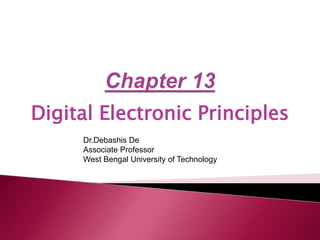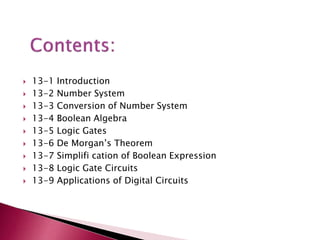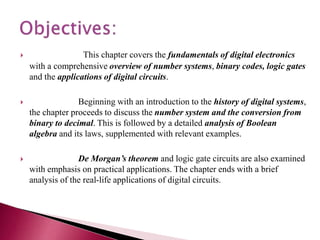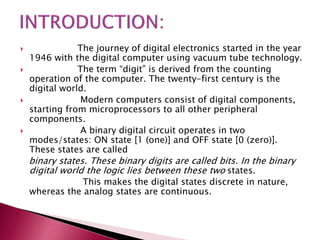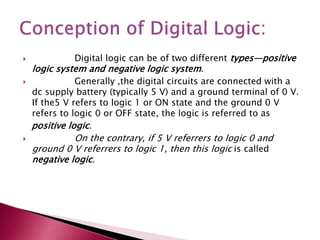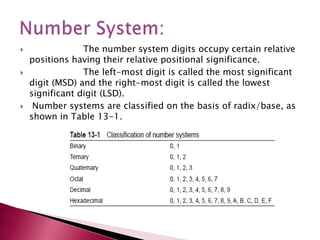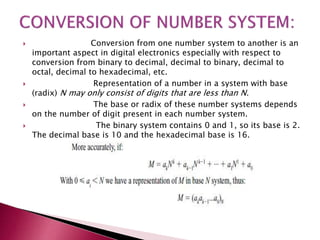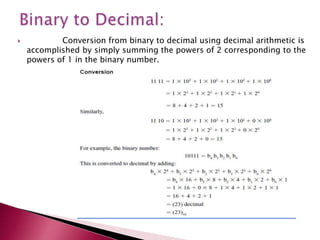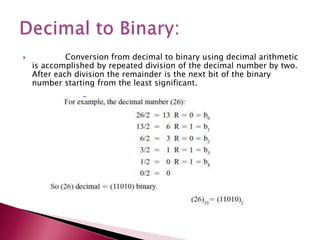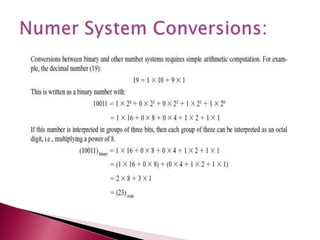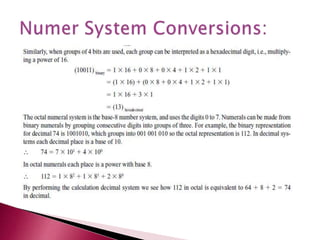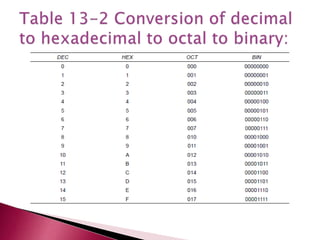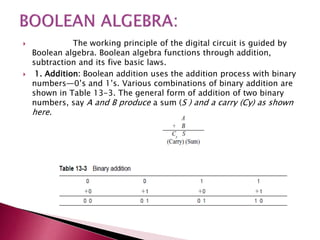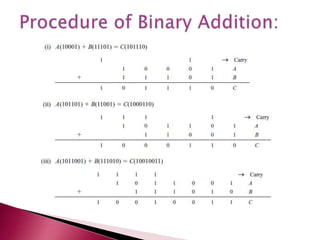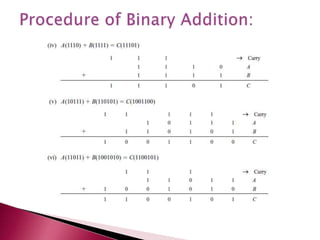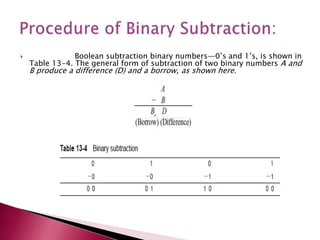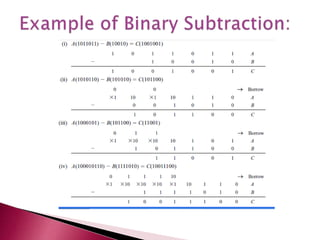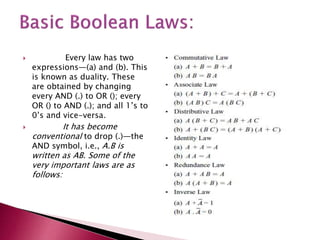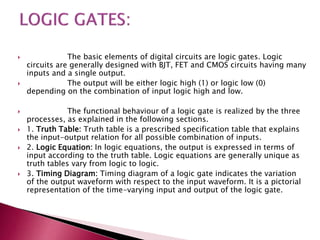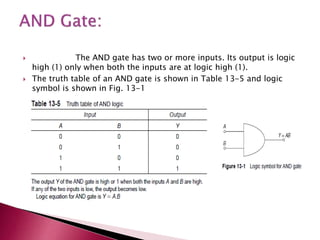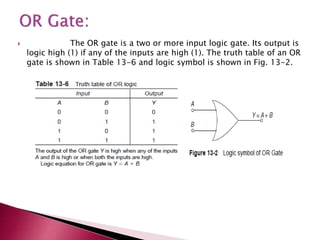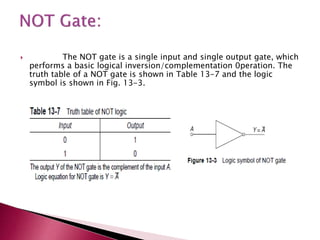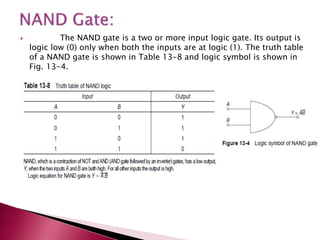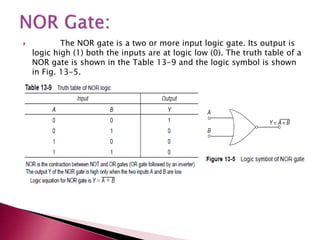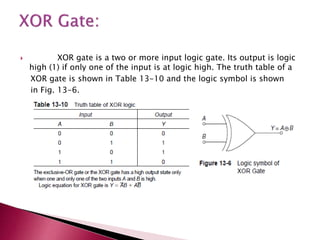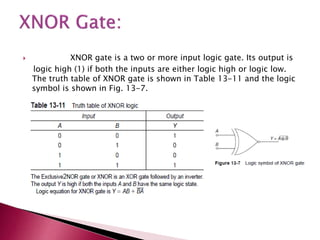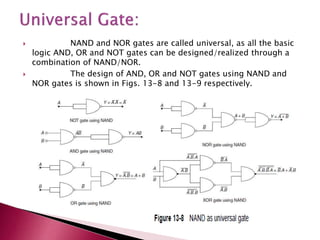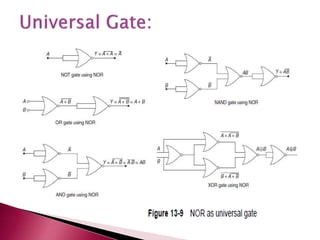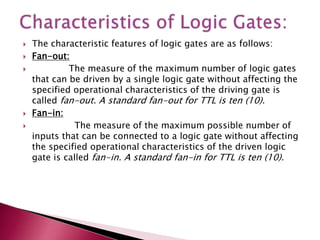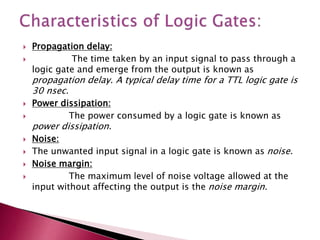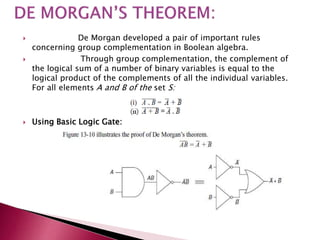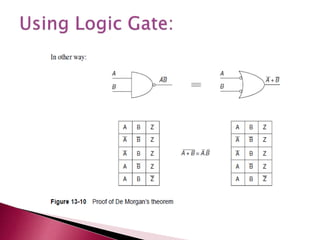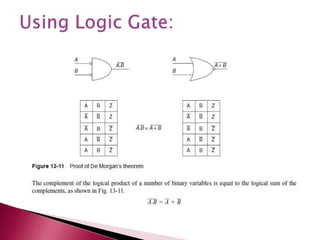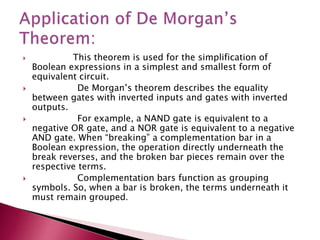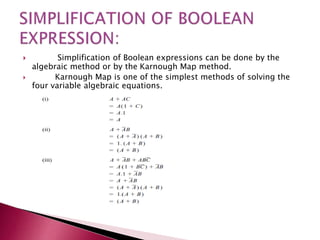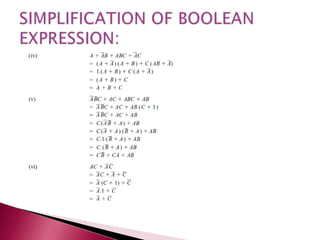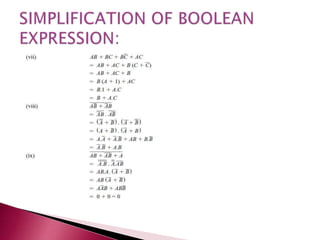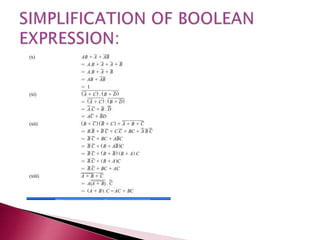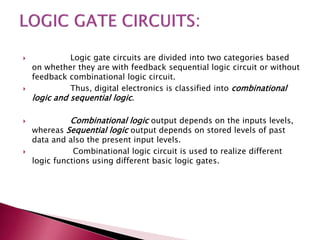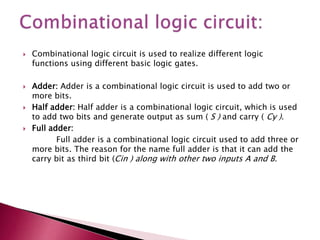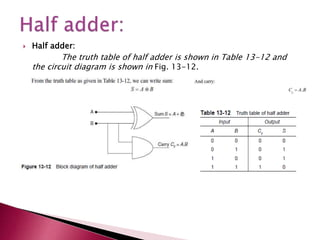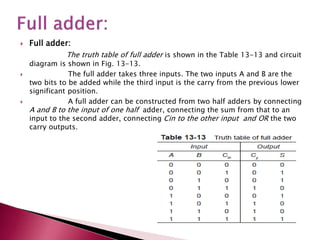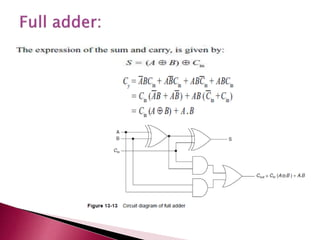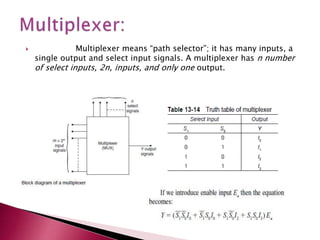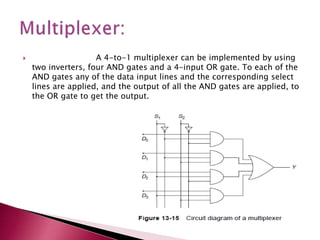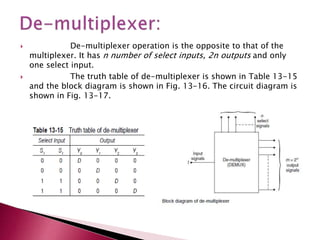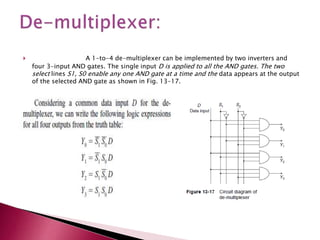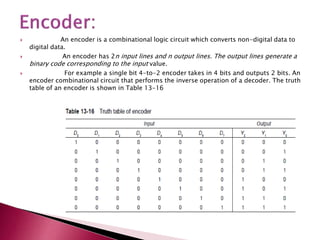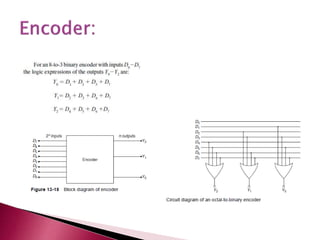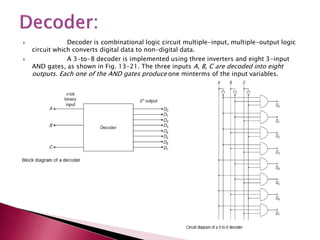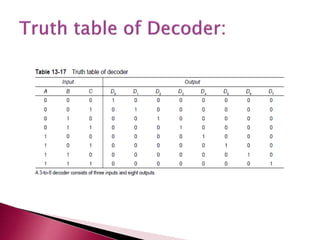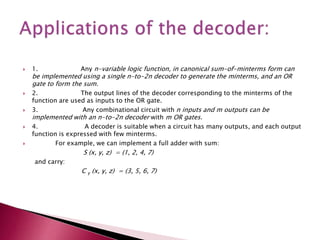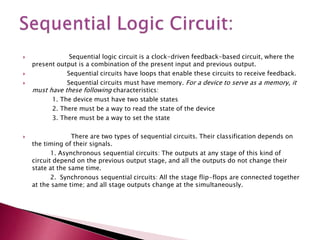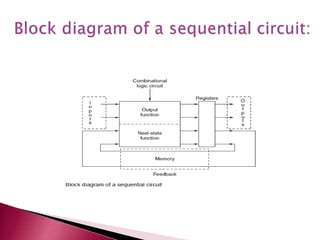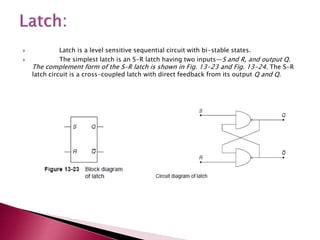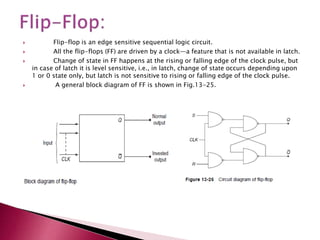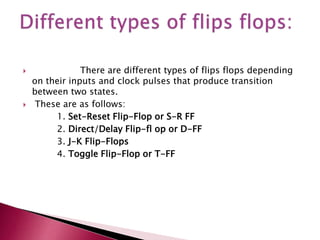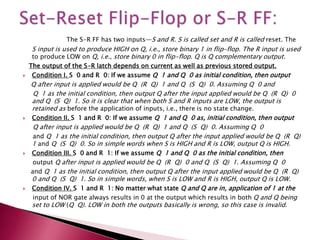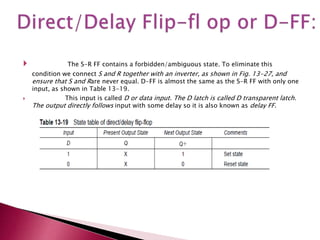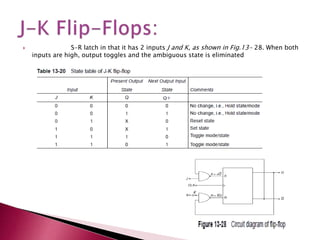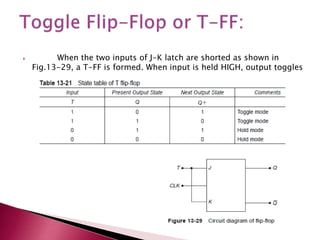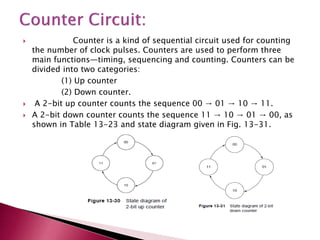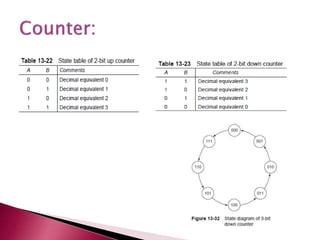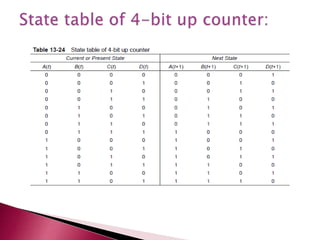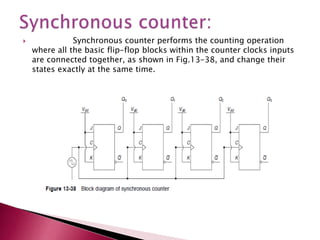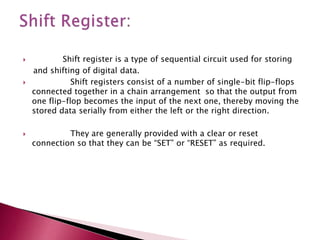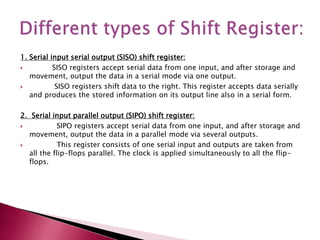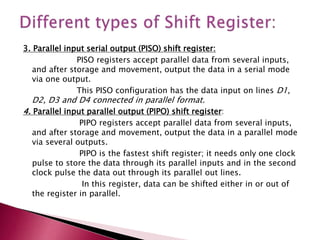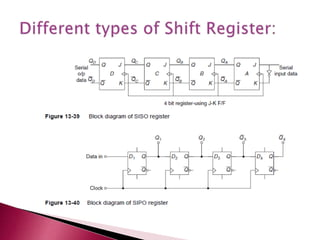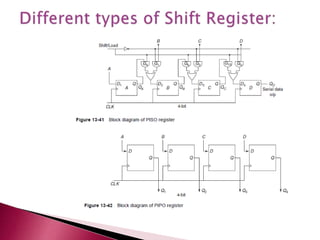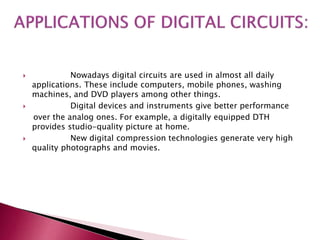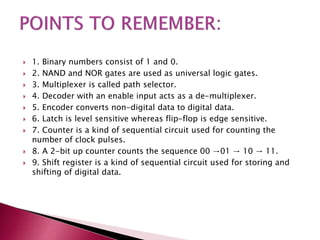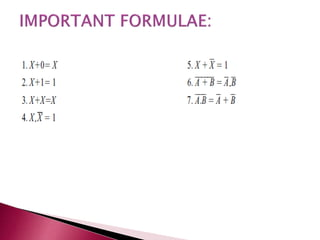1 of 73

### Chapter+13.ppt

1. Digital Electronic Principles Dr.Debashis De Associate Professor West Bengal University of Technology
2.  13-1 Introduction  13-2 Number System  13-3 Conversion of Number System  13-4 Boolean Algebra  13-5 Logic Gates  13-6 De Morgan’s Theorem  13-7 Simplifi cation of Boolean Expression  13-8 Logic Gate Circuits  13-9 Applications of Digital Circuits
3.  This chapter covers the fundamentals of digital electronics with a comprehensive overview of number systems, binary codes, logic gates and the applications of digital circuits.  Beginning with an introduction to the history of digital systems, the chapter proceeds to discuss the number system and the conversion from binary to decimal. This is followed by a detailed analysis of Boolean algebra and its laws, supplemented with relevant examples.  De Morgan’s theorem and logic gate circuits are also examined with emphasis on practical applications. The chapter ends with a brief analysis of the real-life applications of digital circuits.
4.  The journey of digital electronics started in the year 1946 with the digital computer using vacuum tube technology.  The term “digit” is derived from the counting operation of the computer. The twenty-first century is the digital world.  Modern computers consist of digital components, starting from microprocessors to all other peripheral components.  A binary digital circuit operates in two modes/states: ON state [1 (one)] and OFF state [0 (zero)]. These states are called binary states. These binary digits are called bits. In the binary digital world the logic lies between these two states. This makes the digital states discrete in nature, whereas the analog states are continuous.
5.  Digital logic can be of two different types—positive logic system and negative logic system.  Generally ,the digital circuits are connected with a dc supply battery (typically 5 V) and a ground terminal of 0 V. If the5 V refers to logic 1 or ON state and the ground 0 V refers to logic 0 or OFF state, the logic is referred to as positive logic.  On the contrary, if 5 V referrers to logic 0 and ground 0 V referrers to logic 1, then this logic is called negative logic.
6.  The number system digits occupy certain relative positions having their relative positional significance.  The left-most digit is called the most significant digit (MSD) and the right-most digit is called the lowest significant digit (LSD).  Number systems are classified on the basis of radix/base, as shown in Table 13-1.
7.  Conversion from one number system to another is an important aspect in digital electronics especially with respect to conversion from binary to decimal, decimal to binary, decimal to octal, decimal to hexadecimal, etc.  Representation of a number in a system with base (radix) N may only consist of digits that are less than N.  The base or radix of these number systems depends on the number of digit present in each number system.  The binary system contains 0 and 1, so its base is 2. The decimal base is 10 and the hexadecimal base is 16.
8.  Conversion from binary to decimal using decimal arithmetic is accomplished by simply summing the powers of 2 corresponding to the powers of 1 in the binary number.
9.  Conversion from decimal to binary using decimal arithmetic is accomplished by repeated division of the decimal number by two. After each division the remainder is the next bit of the binary number starting from the least significant.
10.  The working principle of the digital circuit is guided by Boolean algebra. Boolean algebra functions through addition, subtraction and its five basic laws.  1. Addition: Boolean addition uses the addition process with binary numbers—0’s and 1’s. Various combinations of binary addition are shown in Table 13-3. The general form of addition of two binary numbers, say A and B produce a sum (S ) and a carry (Cy) as shown here.
11.  Boolean subtraction binary numbers—0’s and 1’s, is shown in Table 13-4. The general form of subtraction of two binary numbers A and B produce a difference (D) and a borrow, as shown here.
12.  Every law has two expressions—(a) and (b). This is known as duality. These are obtained by changing every AND (.) to OR (); every OR () to AND (.); and all 1’s to 0’s and vice-versa.  It has become conventional to drop (.)—the AND symbol, i.e., A.B is written as AB. Some of the very important laws are as follows:
13.  The basic elements of digital circuits are logic gates. Logic circuits are generally designed with BJT, FET and CMOS circuits having many inputs and a single output.  The output will be either logic high (1) or logic low (0) depending on the combination of input logic high and low.  The functional behaviour of a logic gate is realized by the three processes, as explained in the following sections.  1. Truth Table: Truth table is a prescribed specification table that explains the input-output relation for all possible combination of inputs.  2. Logic Equation: In logic equations, the output is expressed in terms of input according to the truth table. Logic equations are generally unique as truth tables vary from logic to logic.  3. Timing Diagram: Timing diagram of a logic gate indicates the variation of the output waveform with respect to the input waveform. It is a pictorial representation of the time-varying input and output of the logic gate.
14.  The AND gate has two or more inputs. Its output is logic high (1) only when both the inputs are at logic high (1).  The truth table of an AND gate is shown in Table 13-5 and logic symbol is shown in Fig. 13-1
15.  The OR gate is a two or more input logic gate. Its output is logic high (1) if any of the inputs are high (1). The truth table of an OR gate is shown in Table 13-6 and logic symbol is shown in Fig. 13-2.
16.  The NOT gate is a single input and single output gate, which performs a basic logical inversion/complementation 0peration. The truth table of a NOT gate is shown in Table 13-7 and the logic symbol is shown in Fig. 13-3.
17.  The NAND gate is a two or more input logic gate. Its output is logic low (0) only when both the inputs are at logic (1). The truth table of a NAND gate is shown in Table 13-8 and logic symbol is shown in Fig. 13-4.
18.  The NOR gate is a two or more input logic gate. Its output is logic high (1) both the inputs are at logic low (0). The truth table of a NOR gate is shown in the Table 13-9 and the logic symbol is shown in Fig. 13-5.
19.  XOR gate is a two or more input logic gate. Its output is logic high (1) if only one of the input is at logic high. The truth table of a XOR gate is shown in Table 13-10 and the logic symbol is shown in Fig. 13-6.
20.  XNOR gate is a two or more input logic gate. Its output is logic high (1) if both the inputs are either logic high or logic low. The truth table of XNOR gate is shown in Table 13-11 and the logic symbol is shown in Fig. 13-7.
21.  NAND and NOR gates are called universal, as all the basic logic AND, OR and NOT gates can be designed/realized through a combination of NAND/NOR.  The design of AND, OR and NOT gates using NAND and NOR gates is shown in Figs. 13-8 and 13-9 respectively.
22.  The characteristic features of logic gates are as follows:  Fan-out:  The measure of the maximum number of logic gates that can be driven by a single logic gate without affecting the specified operational characteristics of the driving gate is called fan-out. A standard fan-out for TTL is ten (10).  Fan-in:  The measure of the maximum possible number of inputs that can be connected to a logic gate without affecting the specified operational characteristics of the driven logic gate is called fan-in. A standard fan-in for TTL is ten (10).
23.  Propagation delay:  The time taken by an input signal to pass through a logic gate and emerge from the output is known as propagation delay. A typical delay time for a TTL logic gate is 30 nsec.  Power dissipation:  The power consumed by a logic gate is known as power dissipation.  Noise:  The unwanted input signal in a logic gate is known as noise.  Noise margin:  The maximum level of noise voltage allowed at the input without affecting the output is the noise margin.
24.  De Morgan developed a pair of important rules concerning group complementation in Boolean algebra.  Through group complementation, the complement of the logical sum of a number of binary variables is equal to the logical product of the complements of all the individual variables. For all elements A and B of the set S:  Using Basic Logic Gate:
25.  This theorem is used for the simplification of Boolean expressions in a simplest and smallest form of equivalent circuit.  De Morgan’s theorem describes the equality between gates with inverted inputs and gates with inverted outputs.  For example, a NAND gate is equivalent to a negative OR gate, and a NOR gate is equivalent to a negative AND gate. When “breaking” a complementation bar in a Boolean expression, the operation directly underneath the break reverses, and the broken bar pieces remain over the respective terms.  Complementation bars function as grouping symbols. So, when a bar is broken, the terms underneath it must remain grouped.
26.  Simplification of Boolean expressions can be done by the algebraic method or by the Karnough Map method.  Karnough Map is one of the simplest methods of solving the four variable algebraic equations.
27.  Logic gate circuits are divided into two categories based on whether they are with feedback sequential logic circuit or without feedback combinational logic circuit.  Thus, digital electronics is classified into combinational logic and sequential logic.  Combinational logic output depends on the inputs levels, whereas Sequential logic output depends on stored levels of past data and also the present input levels.  Combinational logic circuit is used to realize different logic functions using different basic logic gates.
29.  Half adder: The truth table of half adder is shown in Table 13-12 and the circuit diagram is shown in Fig. 13-12.
30.  Full adder: The truth table of full adder is shown in the Table 13-13 and circuit diagram is shown in Fig. 13-13.  The full adder takes three inputs. The two inputs A and B are the two bits to be added while the third input is the carry from the previous lower significant position.  A full adder can be constructed from two half adders by connecting A and B to the input of one half adder, connecting the sum from that to an input to the second adder, connecting Cin to the other input and OR the two carry outputs.
31.  Multiplexer means “path selector”; it has many inputs, a single output and select input signals. A multiplexer has n number of select inputs, 2n, inputs, and only one output.
32.  A 4-to-1 multiplexer can be implemented by using two inverters, four AND gates and a 4-input OR gate. To each of the AND gates any of the data input lines and the corresponding select lines are applied, and the output of all the AND gates are applied, to the OR gate to get the output.
33.  De-multiplexer operation is the opposite to that of the multiplexer. It has n number of select inputs, 2n outputs and only one select input.  The truth table of de-multiplexer is shown in Table 13-15 and the block diagram is shown in Fig. 13-16. The circuit diagram is shown in Fig. 13-17.
34.  A 1-to-4 de-multiplexer can be implemented by two inverters and four 3-input AND gates. The single input D is applied to all the AND gates. The two select lines S1, S0 enable any one AND gate at a time and the data appears at the output of the selected AND gate as shown in Fig. 13-17.
35.  An encoder is a combinational logic circuit which converts non-digital data to digital data.  An encoder has 2n input lines and n output lines. The output lines generate a binary code corresponding to the input value.  For example a single bit 4-to-2 encoder takes in 4 bits and outputs 2 bits. An encoder combinational circuit that performs the inverse operation of a decoder. The truth table of an encoder is shown in Table 13-16
36.  Decoder is combinational logic circuit multiple-input, multiple-output logic circuit which converts digital data to non-digital data.  A 3-to-8 decoder is implemented using three inverters and eight 3-input AND gates, as shown in Fig. 13-21. The three inputs A, B, C are decoded into eight outputs. Each one of the AND gates produce one minterms of the input variables.
37.  1. Any n-variable logic function, in canonical sum-of-minterms form can be implemented using a single n-to-2n decoder to generate the minterms, and an OR gate to form the sum.  2. The output lines of the decoder corresponding to the minterms of the function are used as inputs to the OR gate.  3. Any combinational circuit with n inputs and m outputs can be implemented with an n-to-2n decoder with m OR gates.  4. A decoder is suitable when a circuit has many outputs, and each output function is expressed with few minterms.  For example, we can implement a full adder with sum: S (x, y, z) = (1, 2, 4, 7) and carry: C y (x, y, z) = (3, 5, 6, 7)
38.  Sequential logic circuit is a clock-driven feedback-based circuit, where the present output is a combination of the present input and previous output.  Sequential circuits have loops that enable these circuits to receive feedback.  Sequential circuits must have memory. For a device to serve as a memory, it must have these following characteristics: 1. The device must have two stable states 2. There must be a way to read the state of the device 3. There must be a way to set the state  There are two types of sequential circuits. Their classification depends on the timing of their signals. 1. Asynchronous sequential circuits: The outputs at any stage of this kind of circuit depend on the previous output stage, and all the outputs do not change their state at the same time. 2. Synchronous sequential circuits: All the stage flip-flops are connected together at the same time; and all stage outputs change at the simultaneously.
39.  Latch is a level sensitive sequential circuit with bi-stable states.  The simplest latch is an S-R latch having two inputs—S and R, and output Q. The complement form of the S-R latch is shown in Fig. 13-23 and Fig. 13-24. The S-R latch circuit is a cross-coupled latch with direct feedback from its output Q and Q.
40.  Flip-flop is an edge sensitive sequential logic circuit.  All the flip-flops (FF) are driven by a clock—a feature that is not available in latch.  Change of state in FF happens at the rising or falling edge of the clock pulse, but in case of latch it is level sensitive, i.e., in latch, change of state occurs depending upon 1 or 0 state only, but latch is not sensitive to rising or falling edge of the clock pulse.  A general block diagram of FF is shown in Fig.13-25.
41.  There are different types of flips flops depending on their inputs and clock pulses that produce transition between two states.  These are as follows: 1. Set-Reset Flip-Flop or S-R FF 2. Direct/Delay Flip-fl op or D-FF 3. J-K Flip-Flops 4. Toggle Flip-Flop or T-FF
42. The S-R FF has two inputs—S and R. S is called set and R is called reset. The S input is used to produce HIGH on Q, i.e., store binary 1 in flip-flop. The R input is used to produce LOW on Q, i.e., store binary 0 in flip-flop. Q is Q complementary output. The output of the S-R latch depends on current as well as previous stored output.  Condition I. S 0 and R 0: If we assume Q 1 and Q 0 as initial condition, then output Q after input is applied would be Q (R Q) 1 and Q (S Q) 0. Assuming Q 0 and Q 1 as the initial condition, then output Q after the input applied would be Q (R Q) 0 and Q (S Q) 1. So it is clear that when both S and R inputs are LOW, the output is retained as before the application of inputs, i.e., there is no state change.  Condition II. S 1 and R 0: If we assume Q 1 and Q 0 as, initial condition, then output Q after input is applied would be Q (R Q) 1 and Q (S Q) 0. Assuming Q 0 and Q 1 as the initial condition, then output Q after the input applied would be Q (R Q) 1 and Q (S Q) 0. So in simple words when S is HIGH and R is LOW, output Q is HIGH.  Condition III. S 0 and R 1: If we assume Q 1 and Q 0 as the initial condition, then output Q after input is applied would be Q (R Q) 0 and Q (S Q) 1. Assuming Q 0 and Q 1 as the initial condition, then output Q after the input applied would be Q (R Q) 0 and Q (S Q) 1. So in simple words, when S is LOW and R is HIGH, output Q is LOW.  Condition IV. S 1 and R 1: No matter what state Q and Q are in, application of 1 at the input of NOR gate always results in 0 at the output which results in both Q and Q being set to LOW (Q Q). LOW in both the outputs basically is wrong, so this case is invalid.
43.  The S-R FF contains a forbidden/ambiguous state. To eliminate this condition we connect S and R together with an inverter, as shown in Fig. 13-27, and ensure that S and Rare never equal. D-FF is almost the same as the S-R FF with only one input, as shown in Table 13-19.  This input is called D or data input. The D latch is called D transparent latch. The output directly follows input with some delay so it is also known as delay FF.
44.  S-R latch in that it has 2 inputs J and K, as shown in Fig.13- 28. When both inputs are high, output toggles and the ambiguous state is eliminated
45.  When the two inputs of J-K latch are shorted as shown in Fig.13-29, a T-FF is formed. When input is held HIGH, output toggles
46.  Counter is a kind of sequential circuit used for counting the number of clock pulses. Counters are used to perform three main functions—timing, sequencing and counting. Counters can be divided into two categories: (1) Up counter (2) Down counter.  A 2-bit up counter counts the sequence 00 → 01 → 10 → 11.  A 2-bit down counter counts the sequence 11 → 10 → 01 → 00, as shown in Table 13-23 and state diagram given in Fig. 13-31.
47.  Synchronous counter performs the counting operation where all the basic flip-flop blocks within the counter clocks inputs are connected together, as shown in Fig.13-38, and change their states exactly at the same time.
48.  Shift register is a type of sequential circuit used for storing and shifting of digital data.  Shift registers consist of a number of single-bit flip-flops connected together in a chain arrangement so that the output from one flip-flop becomes the input of the next one, thereby moving the stored data serially from either the left or the right direction.  They are generally provided with a clear or reset connection so that they can be “SET” or “RESET” as required.
49. 1. Serial input serial output (SISO) shift register:  SISO registers accept serial data from one input, and after storage and movement, output the data in a serial mode via one output.  SISO registers shift data to the right. This register accepts data serially and produces the stored information on its output line also in a serial form. 2. Serial input parallel output (SIPO) shift register:  SIPO registers accept serial data from one input, and after storage and movement, output the data in a parallel mode via several outputs.  This register consists of one serial input and outputs are taken from all the flip-flops parallel. The clock is applied simultaneously to all the flip- flops.
50. 3. Parallel input serial output (PISO) shift register: PISO registers accept parallel data from several inputs, and after storage and movement, output the data in a serial mode via one output. This PISO configuration has the data input on lines D1, D2, D3 and D4 connected in parallel format. 4. Parallel input parallel output (PIPO) shift register: PIPO registers accept parallel data from several inputs, and after storage and movement, output the data in a parallel mode via several outputs. PIPO is the fastest shift register; it needs only one clock pulse to store the data through its parallel inputs and in the second clock pulse the data out through its parallel out lines. In this register, data can be shifted either in or out of the register in parallel.
51.  Nowadays digital circuits are used in almost all daily applications. These include computers, mobile phones, washing machines, and DVD players among other things.  Digital devices and instruments give better performance over the analog ones. For example, a digitally equipped DTH provides studio-quality picture at home.  New digital compression technologies generate very high quality photographs and movies.
52.  1. Binary numbers consist of 1 and 0.  2. NAND and NOR gates are used as universal logic gates.  3. Multiplexer is called path selector.  4. Decoder with an enable input acts as a de-multiplexer.  5. Encoder converts non-digital data to digital data.  6. Latch is level sensitive whereas flip-flop is edge sensitive.  7. Counter is a kind of sequential circuit used for counting the number of clock pulses.  8. A 2-bit up counter counts the sequence 00 →01 → 10 → 11.  9. Shift register is a kind of sequential circuit used for storing and shifting of digital data.